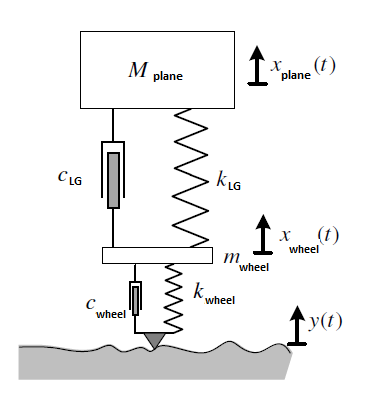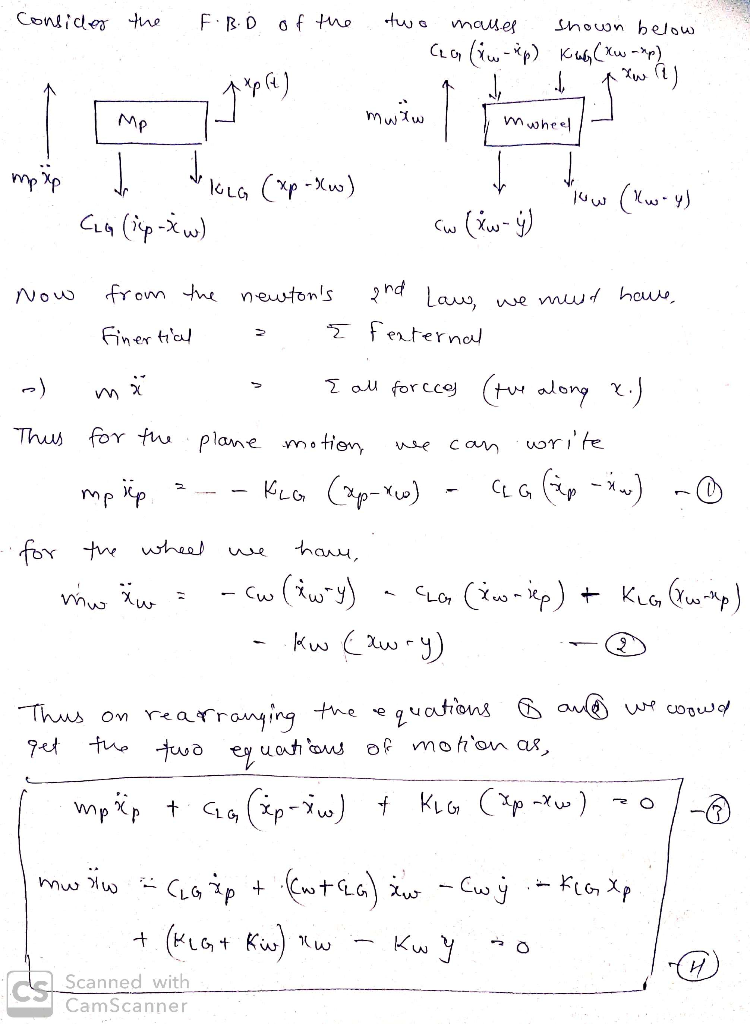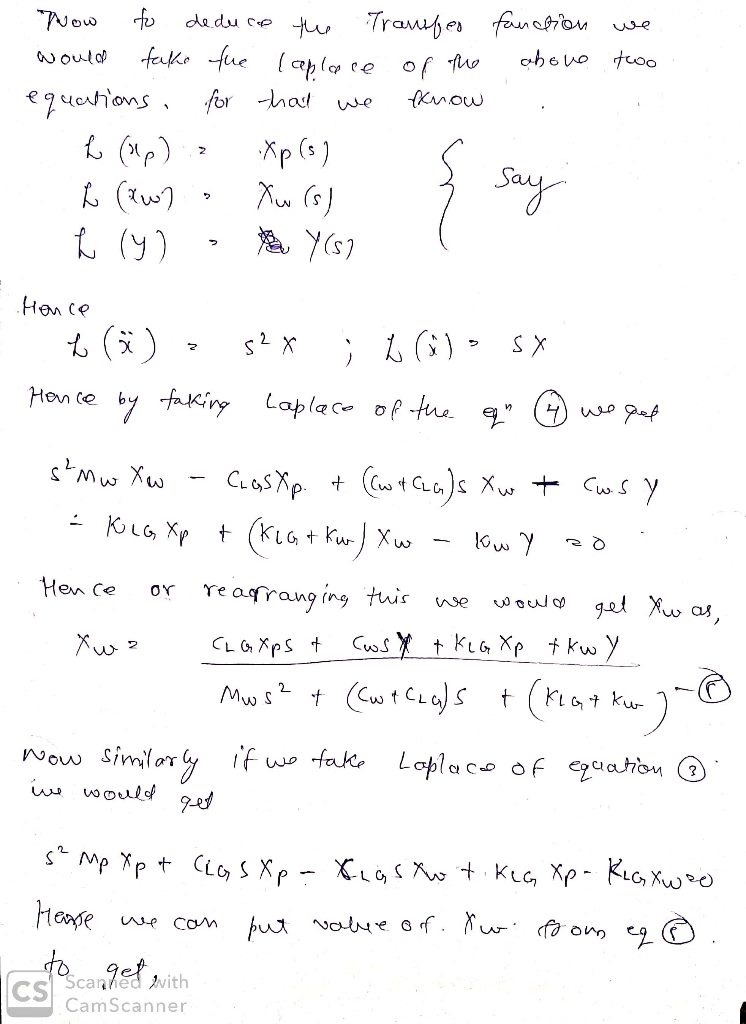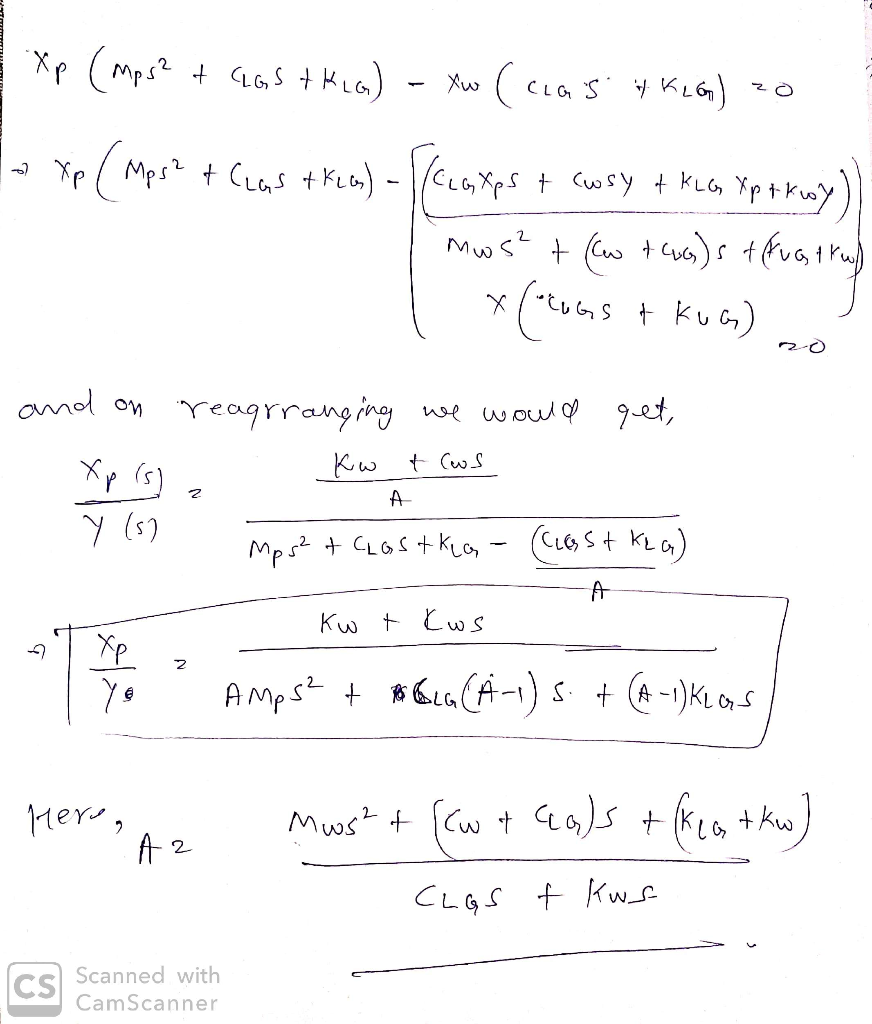# It is a question of studying the dynamic behavior of landing gear (LG) of a F18 landing. To simulate the behavior of the...

```It is a question of studying the dynamic behavior of landing gear (LG) of a F18 landing.
To simulate the behavior of the system we use the figure below.The term 'mwheel' denotes the total mass of the constituent members of the wheel.
We want to know the displacement 'xplane(t)' of the mass 'mplane' for different scenarios.
We consider that the state of the airstrips can be represented by an entry 'y(t)' which simulates the
real geometry of the roadway. The tire is modeled by a linear wheel k and a shock absorber
wheel c.
1. Establish the dynamic equations of system operation;
2. Model the detailed block diagram of the system;
3. Deduce the expression of the transfer function: H(s)= Xplane(s)/Y(s) , the initial conditions
being assumed to be zero;
```
.(t) M plane x plane CLG (t) wheel wheel k. 'wheel wheel y(t) www

In this solution some basic concepts and formulas of Vibration Mechanics are used. For more information, refer to any standard textbook or drop a comment below. Please give a Thumbs Up, if solution is helpful.

Solution :NOTE : Feel free to ask further queries. Your positive rating would be appreciated and motivate me !

##### Add Answer of: It is a question of studying the dynamic behavior of landing gear (LG) of a F18 landing. To simulate the behavior of the...
More Homework Help Questions Additional questions in this topic.